## Example Questions

### Example Question #51 : Rhombuses

If the area of a rhombus is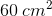, and the length of one of its diagonals is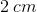, what must be the length of the other diagonal?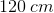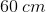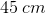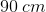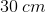Explanation:

Write the formula for the area of a rhombus.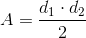Plug in the given area and diagonal length. Solve for the other diagonal.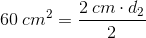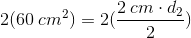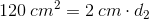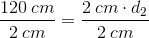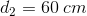### All ACT Math Resources